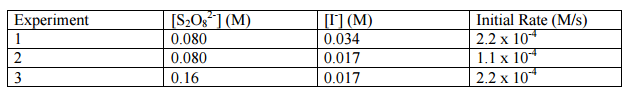# Problem: The reaction of peroxydisulfate ion (S2O8 2- ) with iodide ion (I - ) is: S2O8 2- (aq) + 3I - (aq) → 2SO4 2- (aq) + I3 - (aq) a.) What is the rate law?     b.) What is the rate constant?

###### FREE Expert Solution
92% (214 ratings)
###### Problem Details

The reaction of peroxydisulfate ion (S2O8 2- ) with iodide ion (I - ) is:

S2O8 2- (aq) + 3I - (aq) → 2SO4 2- (aq) + I3 - (aq)

a.) What is the rate law?

b.) What is the rate constant?What scientific concept do you need to know in order to solve this problem?

Our tutors have indicated that to solve this problem you will need to apply the Rate Law concept. You can view video lessons to learn Rate Law. Or if you need more Rate Law practice, you can also practice Rate Law practice problems.

What is the difficulty of this problem?

Our tutors rated the difficulty ofThe reaction of peroxydisulfate ion (S2O8 2- ) with iodide i...as high difficulty.

How long does this problem take to solve?

Our expert Chemistry tutor, Sabrina took 8 minutes and 44 seconds to solve this problem. You can follow their steps in the video explanation above.

What professor is this problem relevant for?

Based on our data, we think this problem is relevant for Professor Dixon's class at UCF.